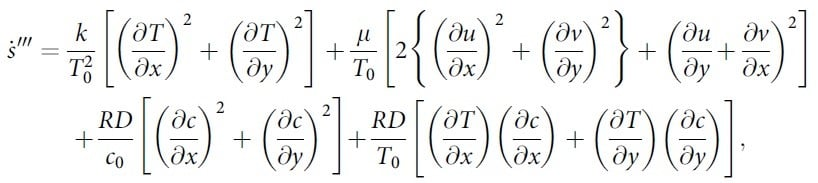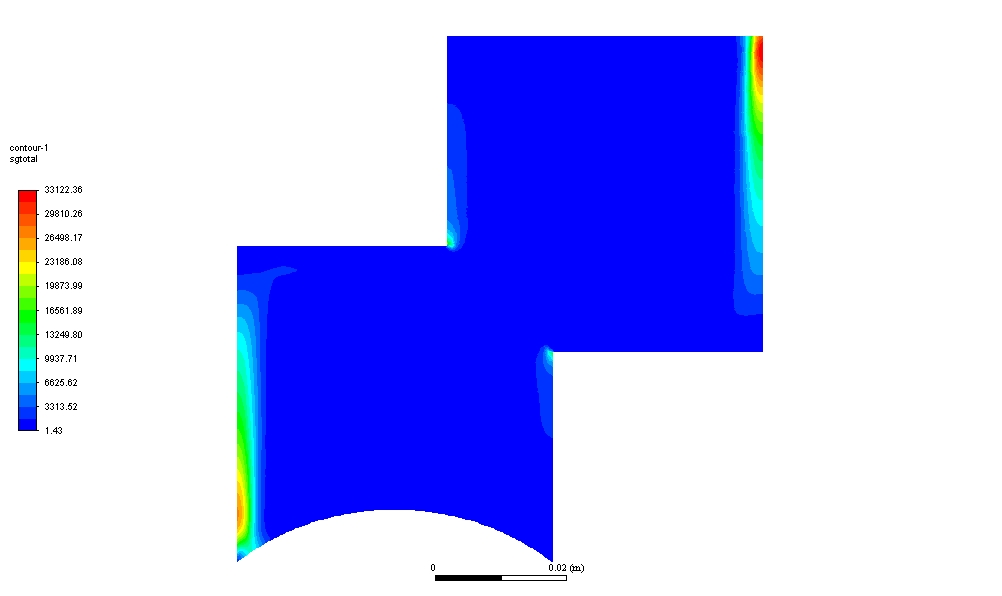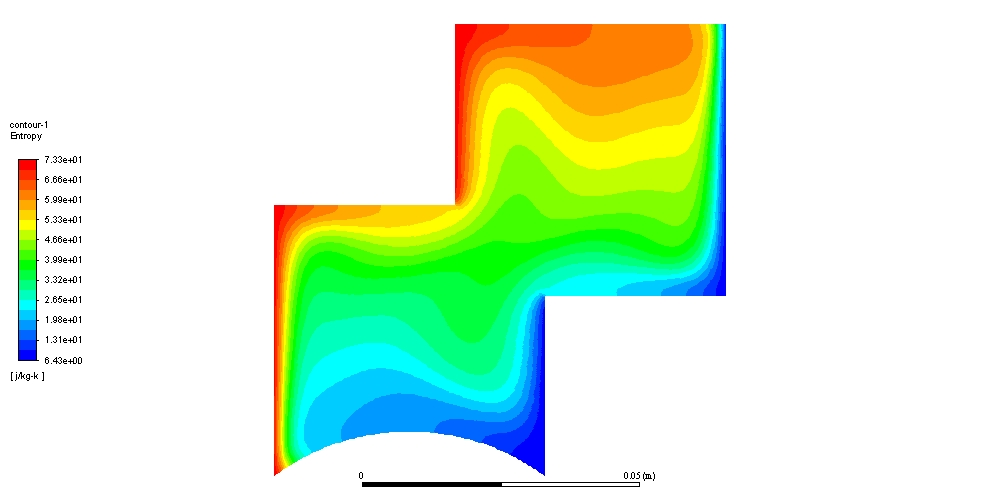## Fluids

•khalidb77
Subscriber

Dear all,

I am trying to evaluate entropy generation by defining the equation below via custom field function within the enclosure below which involve double diffusion and thermal terms in Ansys Fluent 2019:I got a higher entropy generation from the above equations, especially near the walls? may somebody guide me on how I can avoid these higher values near the walls? here is the contour for the total entropy generation I got from the above equation?•Karthik R

Is your solution fully converged? Is this a steady-state result you are looking at?

Thanks.

Karthik

•khalidb77
Subscriber

Thanks Kremella to the answer, yes the solution is converged and all residual are satisfied.  Sorry what did you mean by steady-state result? if you mean that I am simulating steady or transient solution I simulated steady-state solution?

Khalid

•Karthik R

And why do you think that the two inlets (I'm assuming these are inlets) should have lower values of entropy generation?

Thanks.

Karthik

•khalidb77
Subscriber

Dear Kremella,

Thanks for your answer. These all are walls we do not have inlets here.  We think it high because these are contours for entropy generation when compared with respect to Entropy contours that viewed from Temperature there is a big difference between the two parameters. Here is the contour of entropy attached:•Karthik R